#Künstlich Intelligenz #Generator #GAN

# GANs: The creative neural networks

Mag. Dr. Bernhard Mayr, MBA 25.11.2021
#Künstlich Intelligenz#Generator#GAN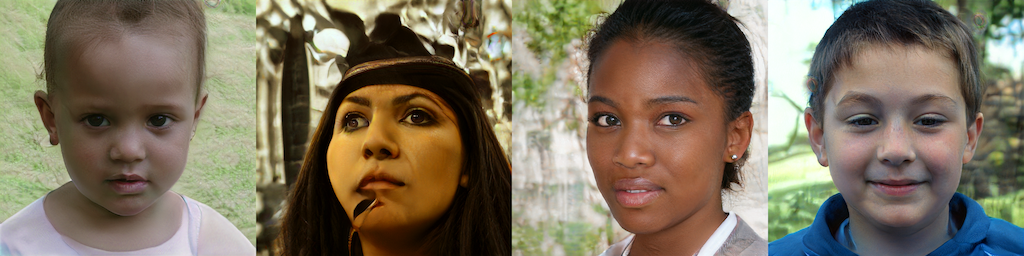You are probably familiar with the idea of using (deep) neural networks as classifiers. The AI-Modell can discriminate different image types - for example: classifying different dog breeds, classifiying images of flowers or herbs, etc.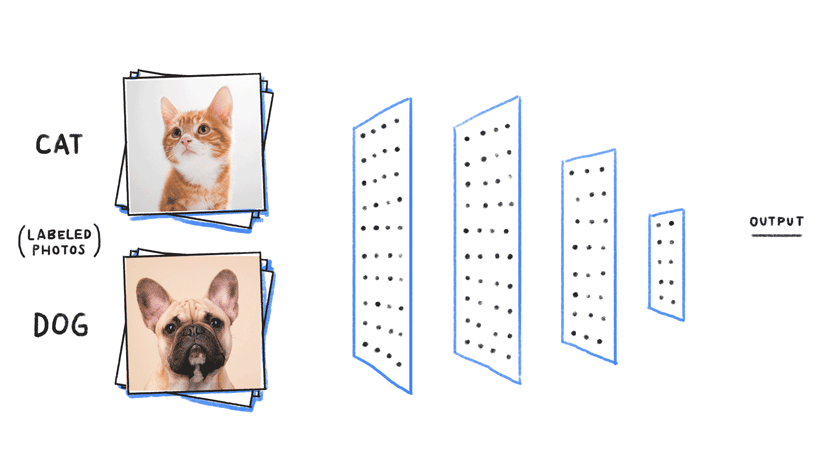More mathematically: the classifier wants to find $p(y|x)$: the probability that given an image input $x$, the image class $y$ is cat, $p(y=\text{cat}|x)$, or dog, $p(y=\text{dog}|x)$.

Now for the neural work to work as a GAN we need two components:

• the creative (generative) part and

## The Discriminator

We have already shown that the discriminator of a GAN model is just a classifier. The same structural component, that we already know from ”classifier AI-Models“.

We will describe the task of the discriminator in a GAN a bit later and now have a look at the Generator

## The Generator

The generator is the “creative part” of the GAN. The generator’s task is to build a description of different classes of elements. We can formulate this job by the help of probabilities:

The generator has to find out $p(x|y)$: the probability that, given that you generated a starfruit $(y=\text{starfruit})$, the resulting image $(x)$ is the one generated. The output space of possible starfruit images is huge, so that makes this challenging for the generator.

While the generator and the discriminator are the counterparts within a GAN, the generator has a job that is way more difficult than the discriminator’s job. This is way the generator needs several steps of improvement for every single step of the discriminator.

The image below shows how the generator tries to figure out those features that represent all cats by the help of the discriminator.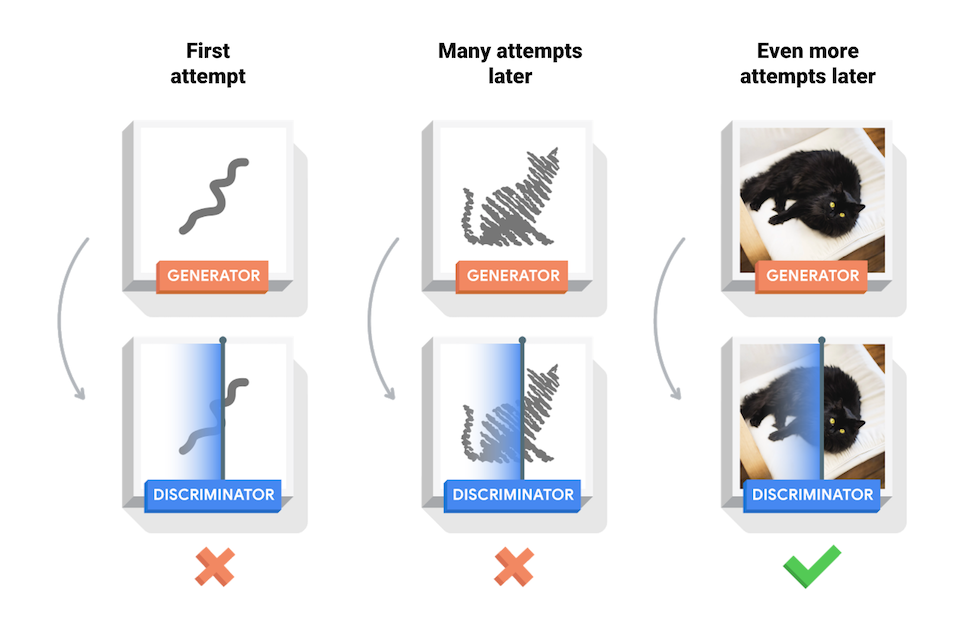Image Credit: TensorFlow

## How the Discriminator helps the Generator to improve

The generator’s first attemps to represent feature values that look like cats / starfruit / chairs / portraits / etc. are just random noise. Now the discriminators job is to decide if the generated image is real or is fake using the training data.

This way the discriminator creates feedback for the generator to improve different feature values and become better in generating artificial images (or text, or …).

## The Noise vector $z$

The noise vector contains some random noise to make sure that the images of a certain class $y$, generated by the generator, don’t look all the same.

The noise vector contains values by sampling random numbers between 0 and 1 uniformly or values from a normal distribution, which you can denote $z$ ~ $N(0, 1)$. This formula describes a normal distribution with a mean value of 0 and a variance of 1.

For real world applications we choose a much larger $z$ to allow for more possible combinations of what $z$ could be. The default values for $z$ is 100. You could also think of using a power of 2 like 128, 512, etc. Nevertheless the number itself doesn’t matter that much. It should just be larger enough to allow a lot of possibilities.

Since we are sampling from a normal distribution, the model will get more values during training within a range of the standard deviation from the mean than values at the tails of the distribution. Therefore the model will get familiar with this range of the noise vector model those areas more likely. Within these areas the model will generate more realistic results. The model is preventing risks of failure for those regions mapped from those familiar regions of the noise vector.

We clearly recognize the trade-off between fidelity (realistic, high quality images) and diversity (variety in images).Image Credit: Modelica

But we can reduce this trade-off with the truncation trick. We are resampling the vector $z$ until it falls within some bound of the normal distribution. In other words: we are resampling $z$ from a truncated normal distribution where we cut off the tails at different values.

The red line shows the truncated normal distribution while the blue line shows the original normal distribution. If we are wiggling these values we are tunig fidelity versus diversity.

For a general use of a GAN-Model we would like to different images for each class, therefore fidelity isn’t always the first priority. A high fidelity means that the model generates one perfect image all of the time. Think of a model that should generate human face images. We wouldn’t like to have a model that just generates one good looking image all of the time.We can also use the StyleGAN Network to generate an interpolation between two images:Or we can use the GAN Model to generate handwritten digits (or characters):

The GAN (generativeadversarial network) architecture allows any artificial intelligence for creativity. Such a model is capable of generating totally new content.

With a GAN you could generate your own virtual product images for publications without the need of a photo shooting and models. Just create totally virtual images. You could use the GAN to generate design ideas for your designers, starting with some requirements.

OpenAI recently released the Dall-E ai-model. This is a model that is able of creating images from text. OpenAI combined capabilities of GPT-3 for text generation tasks and ImageGPT.

Dall-E can perform different tasks combining text analysis and image creation. Perhaps you would like to have an armchair in the shape of an avocado.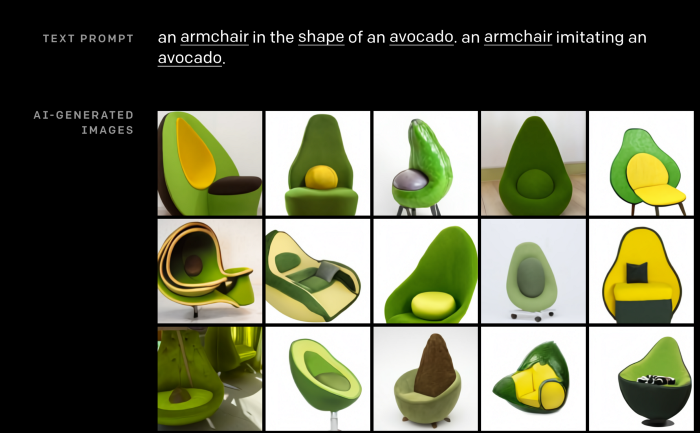Or perhaps you want the model to paint an elephant made of cucumber: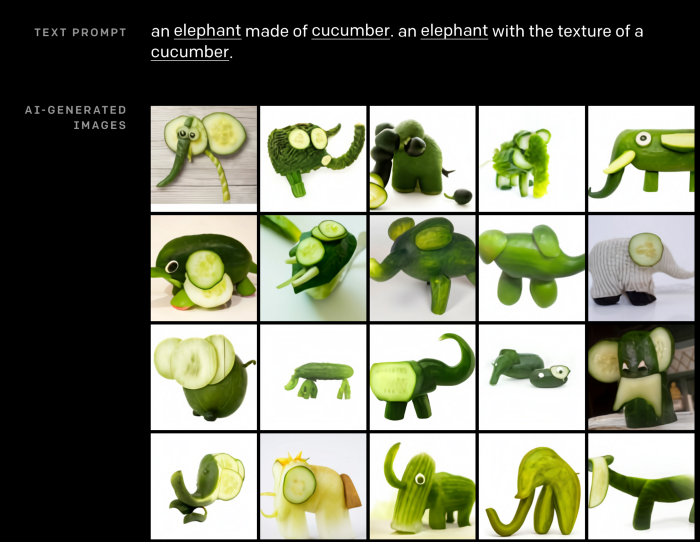Images for the Dall-E are cited from the Dall-E Blog:

← Zurück zum Blog